# VariableImpedance

Multiphase variable impedance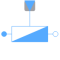# Information

This information is part of the Modelica Standard Library maintained by the Modelica Association.

The impedance model represents a series connection of a resistor and either an inductor or capacitor in each phase.The linear impedance connects the complex voltage `v` with the complex current `i` by `i*Z = v` in each phase, using `m` variable singlephase impedances. The impedances `Z_ref = R_ref + j*X_ref` are given as complex input signals, representing the resistive and reactive components of the input impedances. The resistive components are modeled temperature dependent, so the real part `R_actual = real(Z)` are determined from the actual operating temperatures and the reference input resistances `real(Z_ref)`. Conditional heat ports are considered. The reactive components `X_actual = imag(Z)` are equal to `imag(Z_ref)` if `frequencyDependent = false`. Frequency dependency is considered by `frequencyDependent = true`, distinguishing two cases:

(a) `imag(Z_ref) > 0`: inductive case
The actual reactances `X_actual` are proportional to `f/f_ref`
(b) `imag(Z_ref) < 0`: capacitive case
The actual reactances `X_actual` are proportional to `f_ref/f`

#### Note

Zero crossings of the real or imaginary parts of the impedance signals `Z_ref` could cause singularities due to the actual structure of the connected network.

plug_p plug_n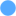Type: PositivePlug Description: Positive quasi-static polyphase plug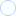Type: NegativePlug Description: Negative quasi-static polyphase plug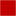Type: HeatPort_a[mh] Description: Conditional heat ports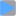Type: ComplexInput[m] Description: Variable complex impedances
v i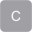Type: ComplexVoltage[m] Description: Complex voltageType: ComplexCurrent[m] Description: Complex current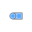Type: PlugToPins_p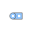Type: PlugToPins_n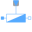Type: VariableImpedance[m]